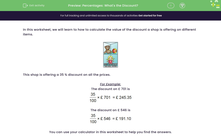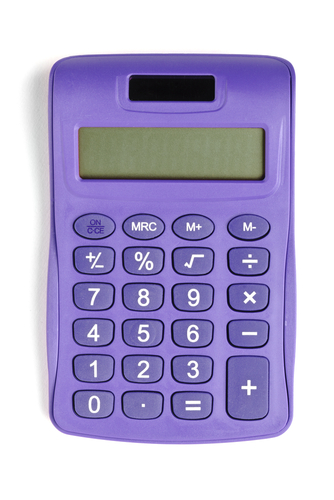# Understand How to Use Percentages to Calculate Discounts

In this worksheet, students will calculate a discount in terms of percentage and amount.Key stage:  KS 2

Curriculum topic:   Ratio and Proportion

Curriculum subtopic:   Solve Percentage Problems

Difficulty level:#### Worksheet Overview

In this activity, we will learn how to calculate the value of the discount a shop is offering on different items.

You may well be used to finding simple percentages by finding 10% first.

For example, to find 40% of £72, you first find 10% which is 7.2.

40% will be 7.2 x 4 = 28.8

So 40% of £72 = £28.80

Sometimes you might need to find a more complicated percentage such as 63%. It is therefore important to know a different method which can be used to find any percentage and this is the method shown below.This shop is offering a 35% discount on all its prices.

For Example:

The discount on £701 is 35% of £701. This is how we write this:The discount on £546 is